### Unitary operators

Suppose we have a basis setthat is complete. These states might be eigenstates of some hermitian operator, say A. If we have another hermitian operator B, then the eigenstates of B could also serve as a complete basis set, lets call it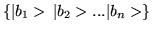. We define the operator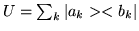, and its adjoint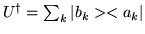. Note that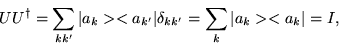(1.46)

and we can show, in the same manner, that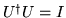. In other words, the adjoint of operator U is its inverse U-1. Operators that have this property are called unitary operators.

Consider any Hilbert space operator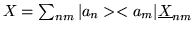where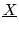is the matrix representation of X with respect to basis. Alternatively, we can express X in terms of thebasis.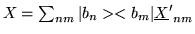where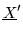is the matrix representation of X in the |bn> basis, or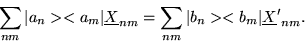(1.47)

We multiply this relation by the bra <al| on the left, and by ket |ap> on the right to get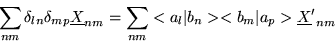(1.48)

but,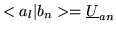and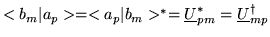or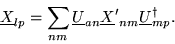(1.49)

In matrix notation this equation is simply written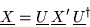(1.50)

that is, operator X, whose matrix representation is given byin the |an> basis, is related to the matrix representation of X in the |bn> basis,, by the above relation. (Note: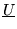is the matrix representation of operator X in the |an> representation).

Matrix equations that have this structure are called similarity transformations, and have important properties. On of such properties is that the eigenvalues of a matrix are invariant under a unitary similarity transformation. That means even though, andare different matrices, their eigenvalues are the same since they are related by a unitary similarity transformation. This implies that we can always findfor any operator representationso that the transformed matrix is diagonal. It's eigenvalues are then distributed on the diagonal.